# How to Create Joint Probability Table in Excel (with Easy Steps)

Get FREE Advanced Excel Exercises with Solutions!

In mathematics, Probability is the measure of the likelihood that an event will occur. Probability is quantified as a number between 0 and 1, where 0 indicates impossibility and 1 indicates certainty. The higher the probability of an event, the more likely it is that the event will occur. Joint Probability is one of a kind. In this article, I will try to explain with step by step procedures how to create joint probability table in Excel. I hope, you have come to the right place if you are looking for the best possible way to create a joint table in Microsoft Excel.

## Introduction to Joint Probability Table

A Joint Probability Table is a mathematical table that shows the probabilities of two or more events occurring together. The table lists all of the possible combinations of events and their corresponding probabilities. It is a powerful tool for statisticians and probability theorists. It can be used to calculate the probability of any combination of events occurring.

## Step-by-Step Procedures to Create Joint Probability Table in Excel

Creation of a Joint Probability Table in Excel is a systematic process. You just need to follow the following procedure to execute the process perfectly.

### Step 1: Create an Organized Dataset

• First of all, arrange the data in a dataset where you can apply probability. In my case, I have decorated a dataset where people play cricket, football, or both. I have arranged them in the Name, Cricket, and Football columns.### Step 2: PivotTable Formation

• Select the entire dataset.
• Next, go to the Insert tab.
• Then, click on From Table/Range from the PivotTable option in the ribbon.• Check the range in the Table/Range section.
• After that, pick your PivotTable location.
• Finally, press OK.• Assign your Rows, Columns, and Values from the PivotTable Fields.Thus, we can have a PivotTable.

### Step 3: Create Joint Probability Table

• Now, copy the entire PivotTable and paste the values in your preferred location.
• You can modify the values according to your choice.

###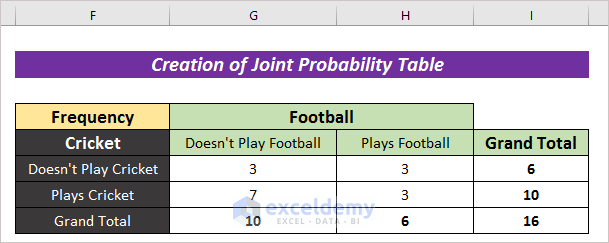• Now, create another copy of the modified table.

###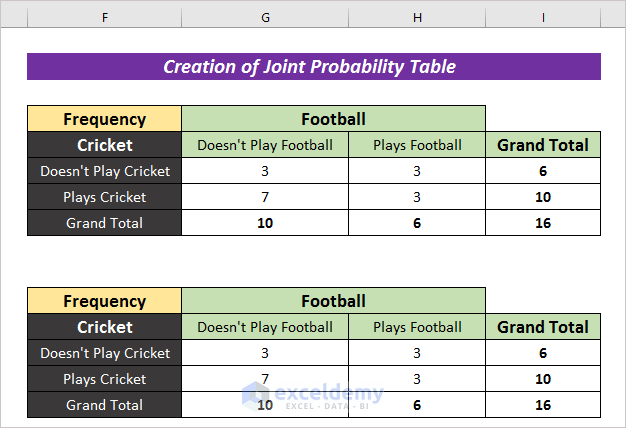• Remove all the values from the new table.

###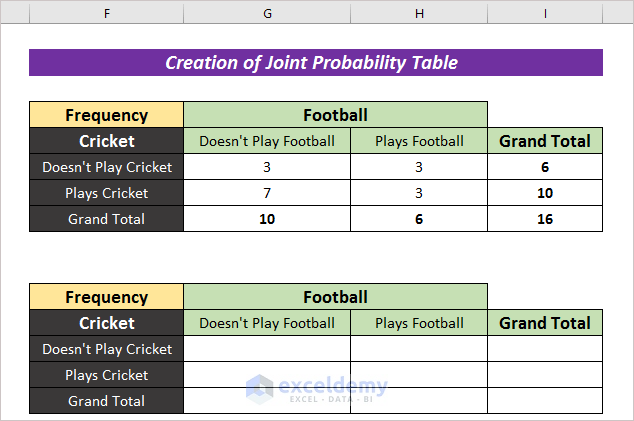• Afterward, input the following formula to convert the whole table with respect to the total value.
`=G6:I8/I8`

###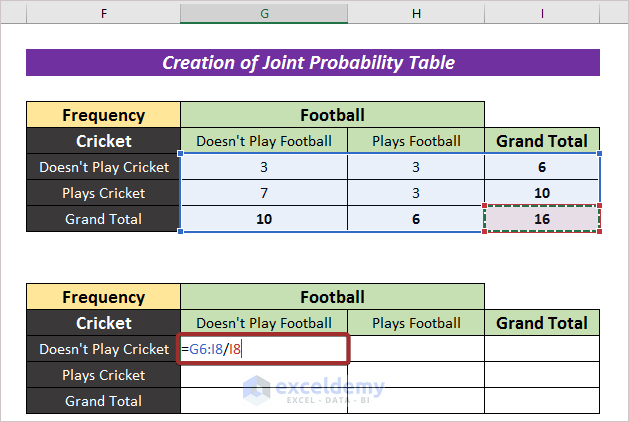• Press ENTER to have the whole value with respect to 1.

###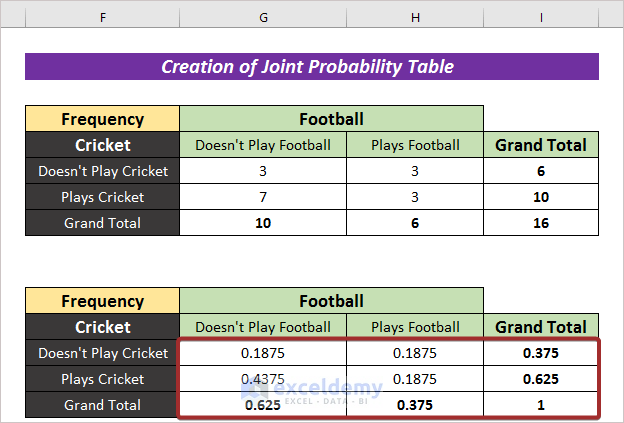• You can change the number format of the table. For this, right-click on the mouse and select Format Cells…

###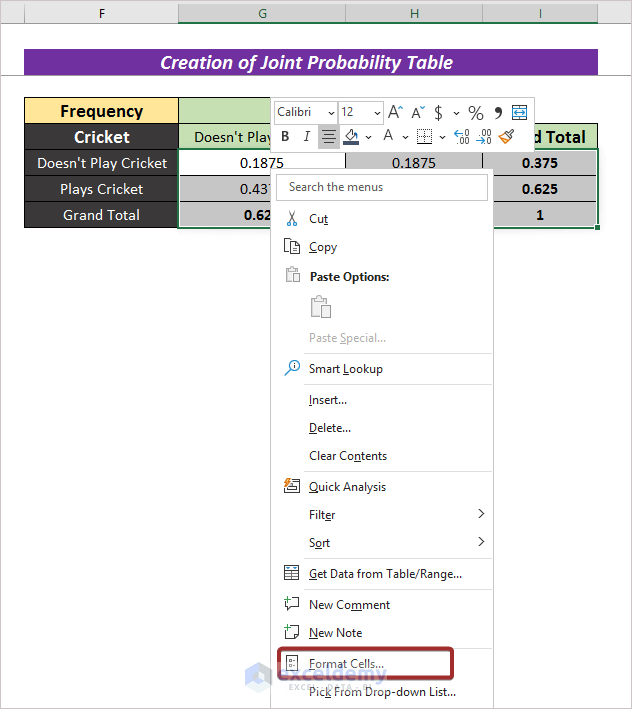A Format Cells wizard will appear.

• From the Category, pick Percentage and click on OK to convert the whole table into percentage format.

###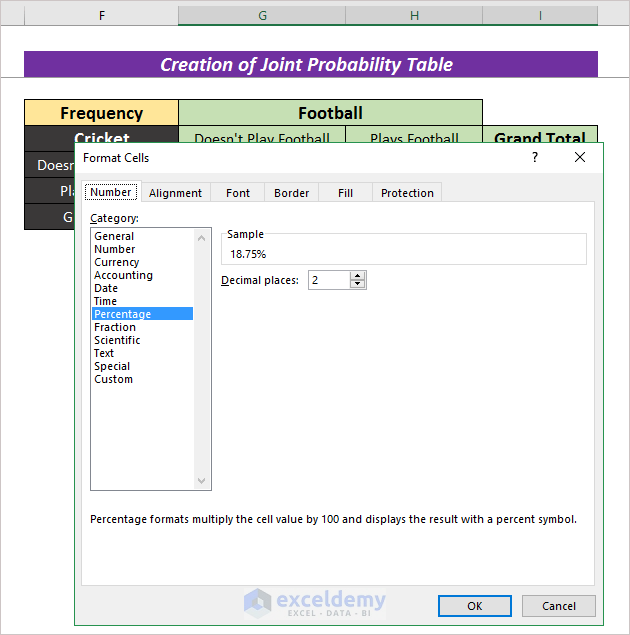Thus, we can have a joint probability table in percentile format.

### Step 4: Different Probabilities Calculation from Joint Probability Table

• For single condition, you can have the value from the probability table. In the following table, we can find the value of people who doesn’t play cricket with the following formula to have the value.
`=I13`• We can use the following formula to have the value where double conditions is satisfied with
`=H13`• To have the value where double conditions is satisfied with OR, apply the following formula:
`=I13+H15-H13`• In order to find the probability of a parameter with respect to another one, divide the intersected value with correspondent parameter. Now, input the formula mentioned below to have the output:
`=G13/I13`## Conclusion

At the end of this article, I like to add that I have tried to explain every detail of how to create Joint Probability Table in Excel. It will be a matter of great pleasure for me if this article could help any Excel user even a little. For any further queries, comment below. You can visit our site for more articles about using Excel.

## Related ArticlesNaimul Hasan Arif

Hello, I am Arif. I am an Engineer who graduated from BUET. I want to pursue an enterprising career in a progressive environment where my skills & knowledge can be enhanced to their maximum potential to contribute to the overall success and growth of the organization.

We will be happy to hear your thoughtsAdvanced Excel Exercises with Solutions PDF